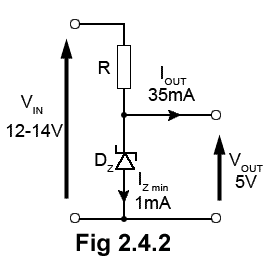# Regulated Power Supplies Quiz

Try this quiz, based on the information you can find in Power Supplies Module 2. Submit your answers and see how many you get right. If you get any answers wrong, just follow the hints to find the right answer, and learn about Regulated Power Supplies as you go.

### 1.Refer to Fig. 2.4.1.What would be the likely output voltage VOUT?

### 2.

Refer to Fig 2.4.1. Which of the following actions would occur if a load resistance connected across the output terminals decreased in value?

### 3.Refer to Fig 2.4.2. What preferred value of resistance would be required for R in order to maintain a minimum zener current (IZ) of 1mA?

### 4.

Which of the following methods would be used to improve the regulation factor of a shunt regulator circuit?

### 5.Refer to Fig 2.4.3. Which of the following statements correctly describes the output voltage VOUT?

### 6.

Refer to Fig 2.4.3. What will be the effect of moving the slider of VR1 downwards?

### 7.

Refer to Fig 2.4.3. What is the purpose of Tr2?

### 8.Refer to Fig 2.4.4. Which of the following components is responsible for sensing the current value?

### 9.

Refer to Fig 2.4.4. What is the purpose of DZ2?

### 10.Refer to Fig. 2.4.5. What is the purpose of D1?

Top of Page